# Kinematics of robot manipulators - forward kinematics 2

## (Denavit Hartenberg method)

If you are unfamiliar with basic principles of robot manipulators I suggest reading the introduction to this series.

This tutorial continues from the previous tutorial which covered forward kinematic analysis using homogeneous transformation matrices. We now examine the Denavit Hartenberg (DH) method for using homogeneous transformation matrices to find the real world co-ordinates of the tool tip where the joint angles are given.  The advantage of the DH method is that only four parameters are required to define transformations, as opposed to six for the previous method (three rotations and three translations). Four input parameters are more economical than six in software terms.

Firstly we explain the DH method and then work through the transformation stages using our robot model as an example.

### Rules for applying the DH method

#### Co-ordinate frames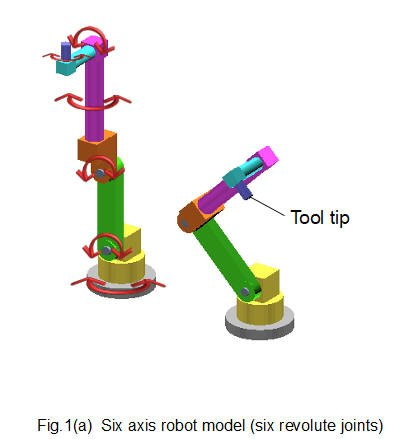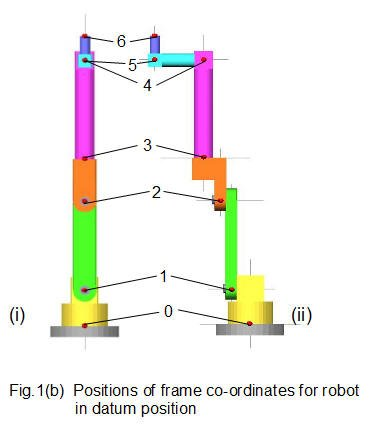Fig.1(a) above shows the six axis robot model used in the previous tutorial, illustrating the vertical datum position and the tool tip pose produced by setting the joint angles.

In the previous tutorial we established co-ordinate frames in the datum position with directions of  X, Y and Z co-ordinates initially the same in each frame.  The first joint and frame were designated #1.

Following convention for the DH method the first co-ordinate frame is designated #0 as shown in Fig.1(b) (i) front and (ii) side views  We also number the joints from #0 to #5 corresponding with the frame number*.  Frame #6 is the tool tip (not a joint). Frame #0 in the datum position is also the base frame in real world co-ordinates.

* It is more conventional to designate the first joint #1 but for this example it seems to me less confusing to use the same number for frame and joint.

The DH method requires co-ordinate frames set up in accordance with very specific rules which we now state.   Co-ordinate frames are considered in pairs, successive frames being designated frame n and frame (n-1) with co-ordinates denoted Xn  X(n-1)  etc.

Rule 1    The axis of rotation of every frame associated with a revolute joint must be the Z axis*.

* also applies to the axis of linear movement for a prismatic joint.

Rule 2    Axis Xn must be perpendicular to axis  Z (n-1)

Rule 3     Axis Xn must intersect axis Z(n-1) .  To achieve this requirement frame n can: (a) be rotated, or (b) translated along one of the joint axes.

Rule 4    The Y axis in all frames must be orientated in accordance with the right hand rule.

#### Setting up co-ordinate frames

We now set up each co-ordinate frame in the robot model in accordance with DH rules.  Diagrams and summaries explain the process.

Fig.2 below illustrates DH rules for frame co-ordinates applied to frame 0 (associated with joint 0) and frame 1 (associated with joint 1).  In this case frame 1 is frame n and frame 0 is frame (n-1)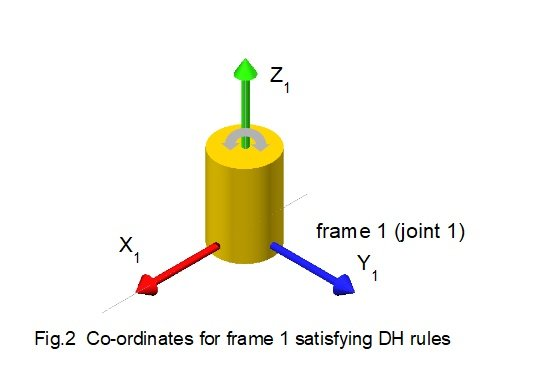Frame 0

• Z0 is the axis of joint rotation
• Rules 2 and 3 do not apply as there is no previous frame
• Y axis corresponds to the right-hand rule

Frame 1

• Z1 is the axis of joint rotation
• Axis X1 is perpendicular to axis Z0
• In its original position at the centre of joint 1 axis X1 does not intersect axis Z0.  By moving frame 1 along the Z1 axis we obtain the necessary intersection shown in Fig.2
• Y axis corresponds to the right-hand rule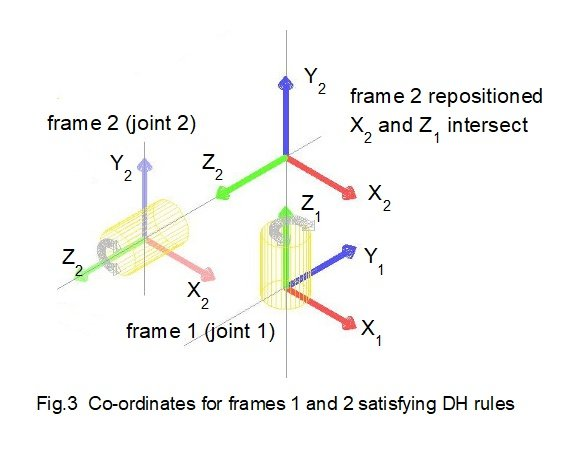Fig.3 above illustrates DH rules for frame co-ordinates applied to frame 1 (associated with joint 1) and frame 2 (associated with joint 2).  In this case frame 2 is frame n and frame 1 is frame (n-1)

Frame 1 has been established (frame repositioned).

Frame 2

• Z2 is the axis of joint rotation
• Axis X2 is perpendicular to axis Z1
• Axis X2 intersects axis Z1
• Y axis corresponds to the right-hand ruleFig.4 above illustrates DH rules for frame co-ordinates applied to frame 2 (associated with joint 2) and frame 3 (associated with joint 3).  In this case frame 3 is frame n and frame 2 is frame (n-1).

Frame 2 has been established.

Frame 3

• Z3 is the axis of joint rotation
• Axis X3 is perpendicular to axis Z2
•  In its original position at the centre of joint 3 axis X3 does not intersect axis Z2.  By moving frame 3 along the Z3 axis we obtain the necessary intersection shown in Fig.4
• Y axis corresponds to the right-hand rule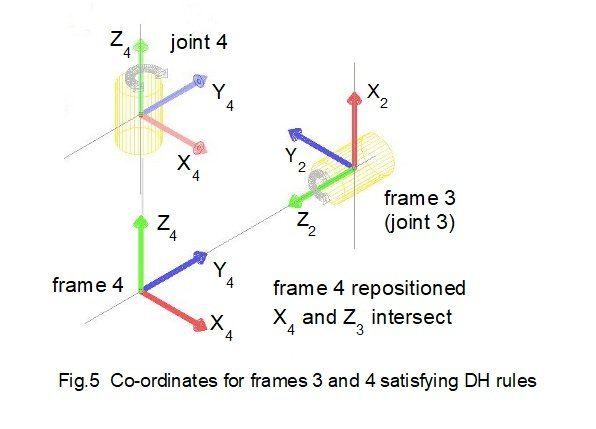Fig.5 above illustrates DH rules for frame co-ordinates applied to frame 3 (associated with joint 3) and frame 4 (associated with joint 4).  In this case frame 4 is frame n and frame 3 is frame (n-1).

Frame 3 has been established (frame repositioned).

Frame 4

• Z4 is the axis of joint rotation
• Axis X4 is perpendicular to axis Z3
• Axis X4 intersects axis Z3
• Y axis corresponds to the right-hand rule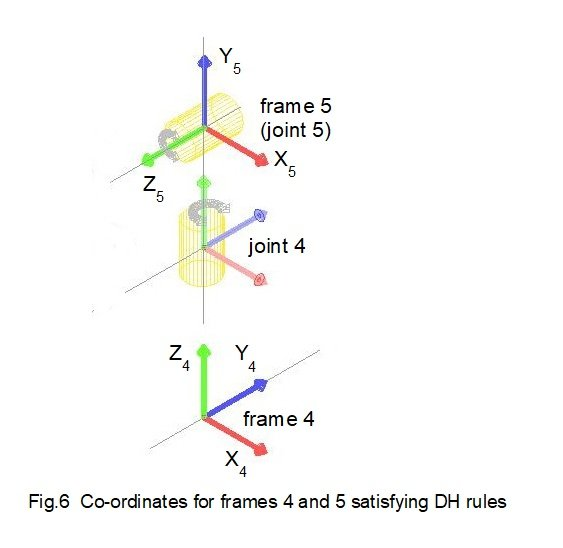Fig.6 above illustrates DH rules for frame co-ordinates applied to frame 4 (associated with joint 4) and frame 5 (associated with joint 5).  In this case frame 5 is frame n and frame 4 is frame (n-1).

Frame 4 has been established.

Frame 5

• Z5 is the axis of joint rotation
• Axis X5 is perpendicular to axis Z4
•  Axis X5 intersects axis Z4
• Y axis corresponds to the right-hand rule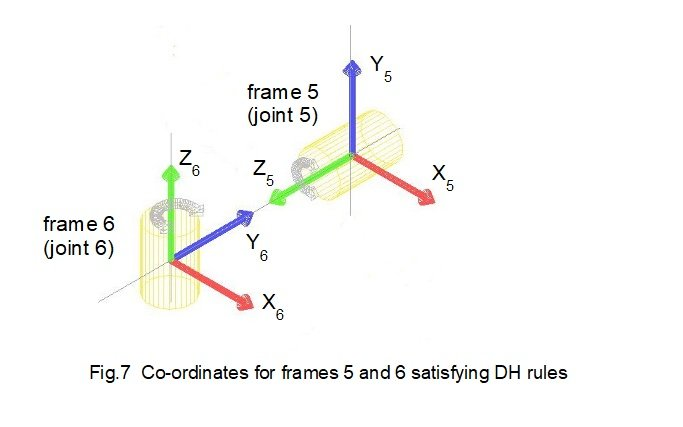Fig.7 above illustrates DH rules for frame co-ordinates applied to frame 5 (associated with joint 5) and frame 6 (associated with the tool tip).  In this case frame 6 is frame n and frame 5 is frame (n-1).

Frame 5 has been established.

Frame 6

• Z6 is the axis of joint rotation
• Axis X6 is perpendicular to axis Z5
• Axis X6 intersects axis Z5
• Y axis corresponds to the right-hand rule

Fig. 8 below shows the complete set of co-ordinate frames for forward kinematic analysis of this six axis robot using the DH method.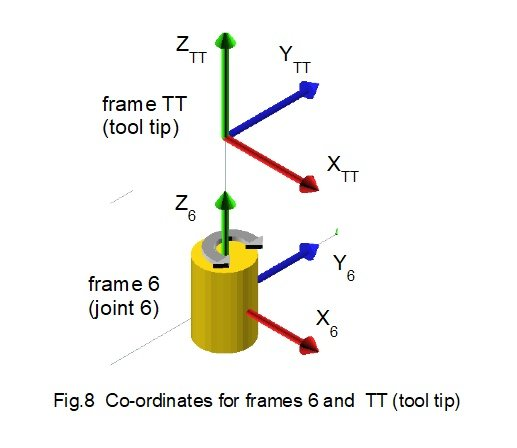#### DH parameters

With the co-ordinate frames set up according to DH rules we now define the four DH parameters designated θ, α (alpha), r * and d.

* sometimes denoted a but easily mistaken in print for α

Parameter θ

θn is the addition of two angles: angle1 is the angle required to rotate axis X(n-1) around axis Z(n-1)  such that axis X(n-1) is parallel to axis X; angle 2 is the angle the revolute joint associated with frame (n-1) rotates around axis Z(n-1)  We will denote angle 1 as θn/ and angle 2 as θn//.

Parameter α

αn is the angle required to rotate axis Z(n-1) around axis* Xn  such that axis Z(n-1) is parallel to axis Zn

* This is a tricky parameter as rotation is around an axis in another frame.

Parameter r

rn is the displacement in the Xn direction from the centre of frame (n -1) to the centre of frame n .

Parameter d

dn is the displacement in the Z(n-1) direction from the centre of frame (n -1) to the centre of frame n .

These parameters will become clearer as we work through each pair of co-ordinate frames.  Note that rotations around and displacements along the Y axis play no active part in the DH method.

DH parameters are normally presented in a table shown below with each co-ordinate pair designated by a number n.  Our robot model has 6 co-ordinate pairs (seven frames*) giving the following parameter table.

* to apply the DH method there must be at least one more frame than there are joints, including one frame at the tool tip / end effector.

 Frames n θ α r d 0, 1 1 1, 2 2 2, 3 3 3, 4 4 4, 5 5 5, 6 6

### DH homogeneous transformation matrix

In the previous tutorial we derived homogeneous transformation matrices T combining:

•  rotation θ  of co-ordinate frame X0, ,Y0 , Z0 about axes X0, ,Y0 ,Z0 to create a new frame X1 ,Y1 ,Z1
• translation of the origin of frame  X1 Y1 Z1 to a position defined by vector [x10, y10, z10].

For rotation about X, Y and Z axes respectively the transformation matrices are: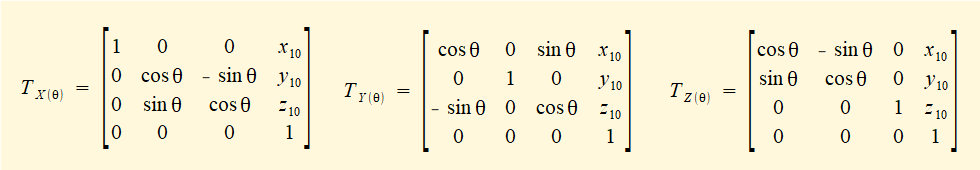The DH transformation matrix is the combination of two rotations and two displacements for designated axes in the DH co-ordinate frames.  Examining the criteria for DH parameters it is seen that the applicable rotation axes are:

• Z(n-1) for which the parameter is θn
• Xn for which the parameter is αn

and the applicable displacement axes are:

•  Z(n-1) for which the parameter is dn
•  Xn for which the parameter is rn

The DH transformation matrix T(n-1)n is a serial combination of the following homogeneous transformation matrices (D denotes a transformation matrix for displacement and R for rotation; the notation is replicated from above).

T(n-1)n = DZ(n-1)(dn) . RZ(n-1)n) . DXn(rn) . RXnn) which gives: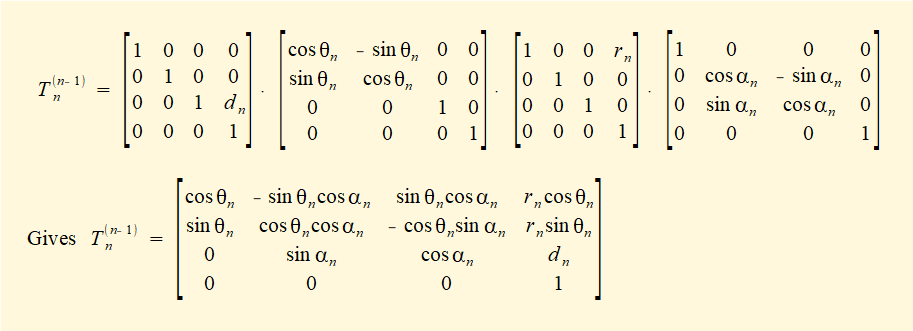We compute this matrix for each paired value of frames n and (n-1) using values of θn, αn , rn and dn from the parameter table.

We now examine each pair of co-ordinate frames to determine the DH parameters.

Referring back to the robot model in Fig.1(a) the settings for joint angles are as follows:

 Joint # Joint angle 0 0 ° 1 45° 2 -90° 3 0° 4 -90° 5 0°

Pair 1 - frame 0 and frame 1 (n = 1)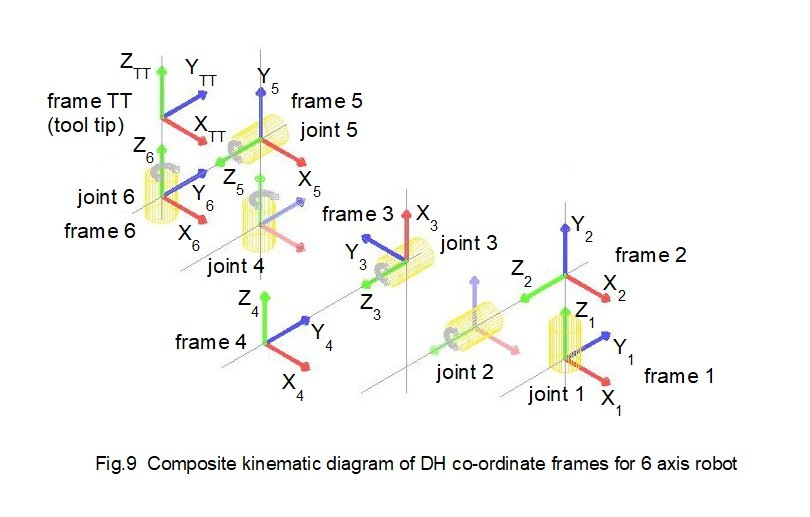Fig.9(a) above shows frame 0 and frame 1 set up using the DH rules (as Fig.2).   Frame 1 is frame n and frame 0 is frame (n-1)  Consider each DH parameter in turn.

Parameter θ1.

θ/1 is the angle required to rotate axis X0 around axis Z0  such that axis X0 is parallel to axis X.  Fig.9(a) shows X0 is already parallel to X1.  Thus θ/1 = 0°.

θ//1  is the angle revolute joint 0 rotates around axis Z0   .  In this case θ//1 = 0°.

Thus  θ1 =  θ/1  +  θ//1  = 0°

Parameter α1

α1 is the angle required to rotate axis Z0 around axis X1  such that axis Z0 is parallel to axis Z1 .  Fig.9(b) shows Z0 must be rotated 90° counter-clockwise around X1 for Z0 to be parallel to Z1.  Thus α1 = 90°.

Parameter r1

r1  is the displacement in the X1 direction from the centre of frame 0 to the centre of frame 1.  Fig9(a) shows there is no displacement between the frame centres in the X1 direction.  Thus  r1 = 0.

Parameter d1

d1 is the displacement in the Z0 direction from the centre of frame 0 to the centre of frame 1.as shown on Fig.9(a).   From the robot model d1 = 160 *.

*  length units are not specified.

The first row of the DH parameter table is now complete.

 Frames n θ α r d 0, 1 1 0° 90° 0 160

We compute the DH transformation matrix T01 to give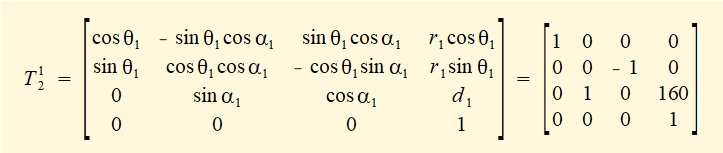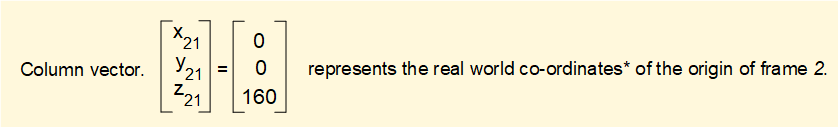* For DH analysis we use the co-ordinates of frame 0 for the base frame.  Thus in this example the Z axis is the real world vertical axis.

The real world co-ordinates of frame 1 are not the real world co-ordinates of the centre of joint 1 because frame 1 was translated along the Z1 axis to satisfy DH rule 3 for setting up frame co-ordinates.

Pair 2 - frame 1 and frame 2 (n = 2)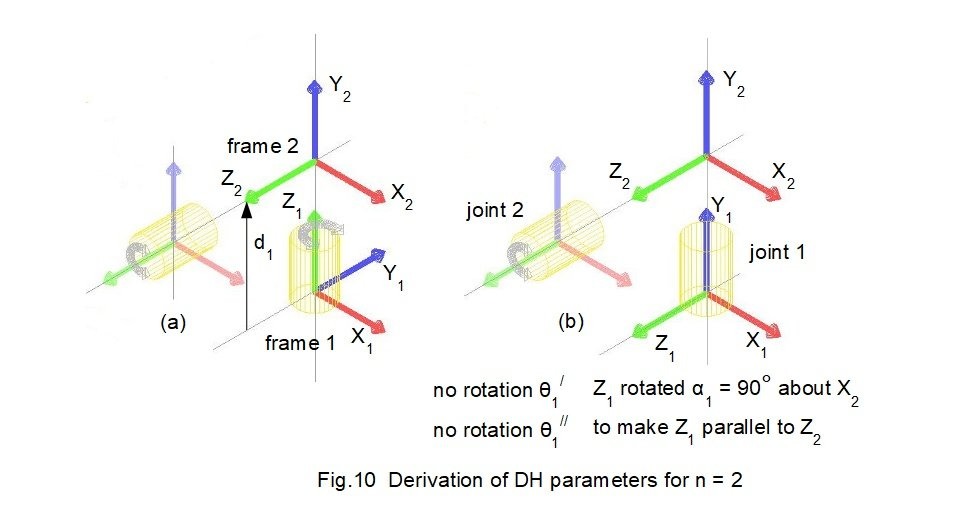Fig.10(a) above shows frame 1 and frame 2 set up using the DH rules (as Fig.3).   Frame 2 is frame n and frame 1 is frame (n-1)  Consider each DH parameter in turn.

Parameter θ2

θ/2 is the angle required to rotate axis X1 around axis Z1  such that axis X1 is parallel to axis X.  Fig.10(b) shows X1 must be rotated 90° counter-clockwise around Z1 to be parallel to X2.  Thus θ/2 = 90°.

θ//2  is the angle revolute joint 1 rotates around axis Z.  In this example θ//2 = 45°.

Thus  θ2 =  θ/2  +  θ//2  = 90° + 45° = 135°

Parameter α2

α2 is the angle required to rotate axis Z1 around axis X2  such that axis Z1 is parallel to axis Z2 .  Fig.10(a) shows Z1 is already parallel to Z2.  Thus α2 = 0°.

Parameter r2

r2 is the displacement in the X2 direction from the centre of frame 1 to the centre of frame 2 as shown on Fig.10(a)  From the robot model, r2 = 400.

Parameter d2

d2 is the displacement in the Z1 direction from the centre of frame 1 to the centre of frame 2 as shown on Fig10(a).   From the robot model d2 = 135.

The second row of the DH parameter table is now complete.

 Frames n θ α r d 0, 1 1 0° 90° 0 160 1, 2 2 135° 0° 400 135

We compute the DH transformation matrix T12 to give* :

* For the remaining transformations we omit this detail.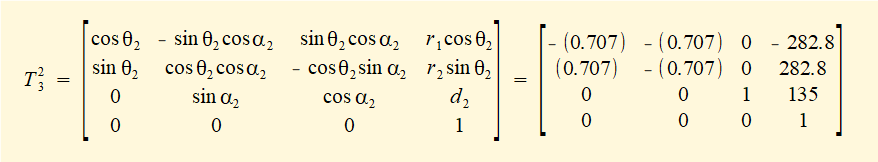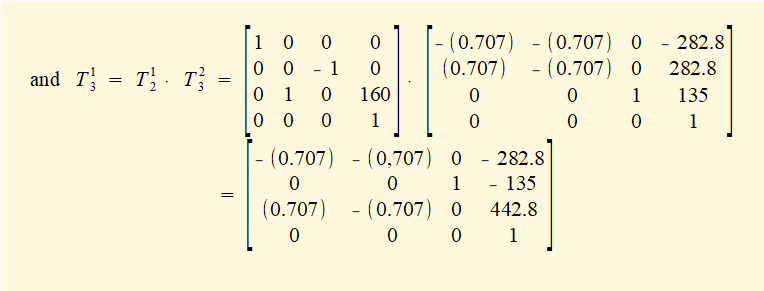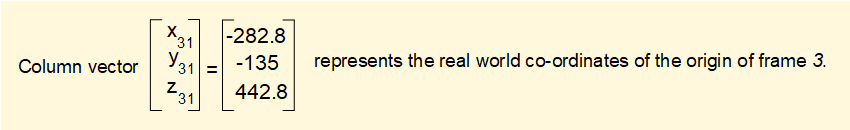Pair 3 - frame 2 and frame 3 (n = 3)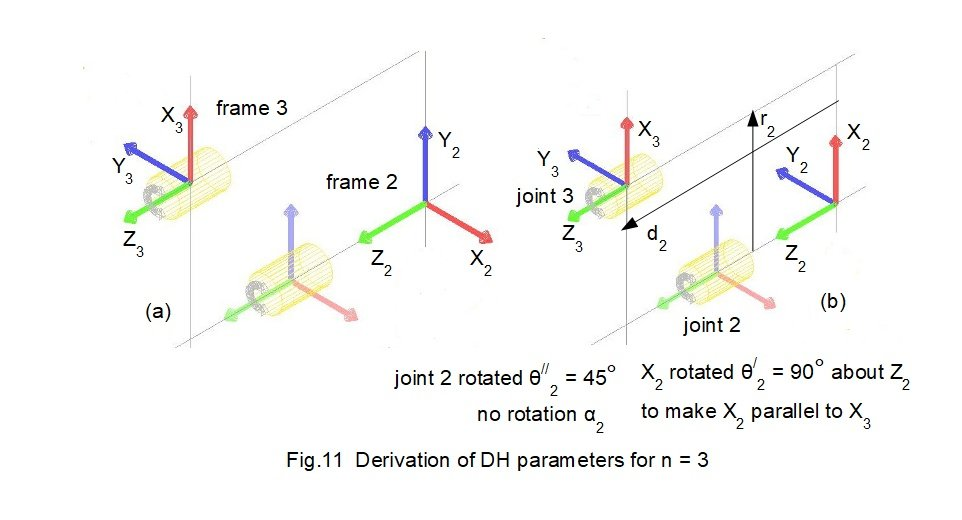Fig.11(a)  above shows frame 2 and frame 3 set up using the DH rules (as Fig.4).   Frame 3 is frame n and frame 2 is frame (n-1)  Consider each DH parameter in turn.

Parameter θ3

θ/3 is the angle required to rotate axis X2 around axis Z2  such that axis X2 is parallel to axis X.  Fig.11(b) shows X2 must be rotated 90° clockwise around Z2 to be parallel to X3.  Thus θ/3 = -90°.

θ//3  is the angle revolute joint 2 rotates around axis Z2 .  In this example θ//3 = -90°.

Thus  θ3 =  θ/3  +  θ//3  = -90° + (-90°) = -180°

Parameter α3

α3 is the angle required to rotate axis Z2 around axis X3  such that axis Z2 is parallel to axis Z3 .   Fig.11(b) shows Z2 must be rotated 90° clockwise around X3 to be parallel to Z3.  Thus α3 = -90°.

Parameter r3

r3 is the displacement in the X2 direction from the centre of frame 2 to the centre of frame 3 as shown on Fig.11(a)  In this case r3 = 0.

Parameter d3

d3 is the displacement in the Z2 direction from the centre of frame 2 to the centre of frame 3 as shown on Fig11(a).   From the robot model, d3 = 75.

The third row of the DH parameter table is now complete.

 Frames n θ α r d 0, 1 1 0° 90° 0 160 1, 2 2 135° 0° 400 135 2, 3 3 -180° -90° 0 75

We compute the DH transformation matrix T23 to give: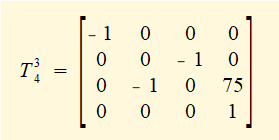and then compute T03 = T02 . T23 giving: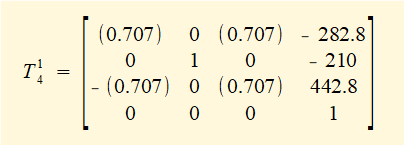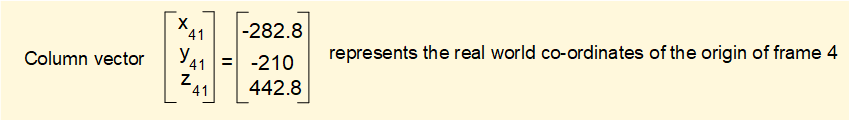Note that the real world co-ordinates of frame 3 are not the co-ordinates of the centre of joint 3 because frame 3 is located to meet DH rule 3 for setting up frame co-ordinates.

Pair 4 - frame 3 and frame 4 (n = 4)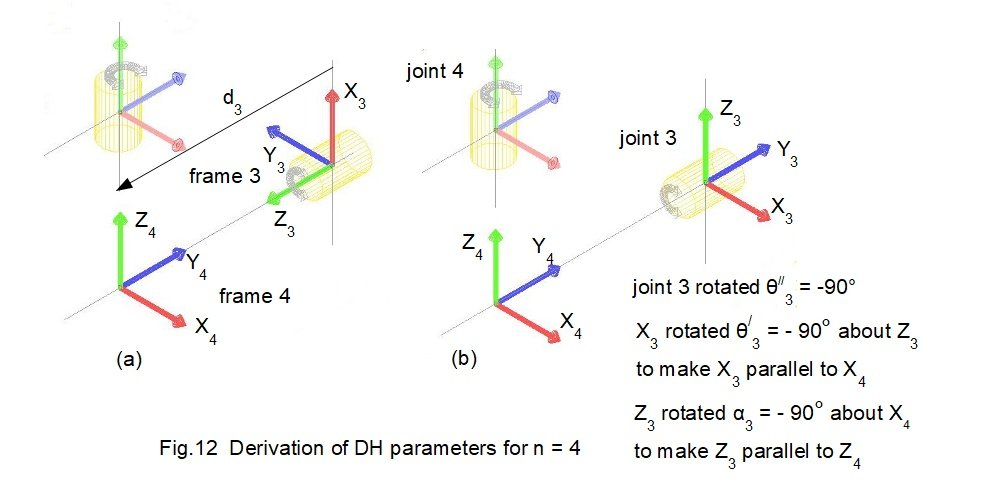Fig.12(a) above shows frame 3 and frame 4 set up using the DH rules (as Fig.5).   Frame 4 is frame n and frame 3 is frame (n-1)  Consider each DH parameter in turn.

Parameter θ4

θ/4 is the angle required to rotate axis X3 around axis Z3  such that axis X3 is parallel to axis X. Fig.12(a) shows X3 is already parallel to X4.  Thus θ/4 = 0°.

θ//4  is the angle revolute joint 3 rotates around axis Z3   .  In this example θ//4 = 0°.

Thus  θ4 =  θ/4  +  θ//4  = -0° + 0° = 0°

Parameter α4

α4 is the angle required to rotate axis Z3 around axis X4  such that axis Z3 is parallel to axis Z4 .   Fig.12(b) shows Z3 must be rotated 90° counter-clockwise around X4 to be parallel to Z4.  Thus α4 = 90°.

Parameter r4

r4 is the displacement in the X3 direction from the centre of frame 3 to the centre of frame 4 as shown on Fig.12(a).  In this case r4 = 0.

Parameter d4

d4 is the displacement in the Z3 direction from the centre of frame 3 to the centre of frame 4 as shown on Fig12(a).   From the robot model, d4 = 630.

The fourth row of the DH parameter table is now complete.

 Frames n θ α r d 0, 1 1 0° 90° 0 160 1, 2 2 45° 0° 400 135 2, 3 3 -180° -90° 0 75 3, 4 4 0° 90° 0 630

We compute the DH transformation matrix T34 to give: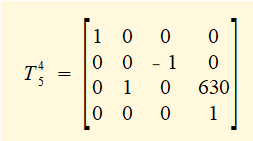and then compute T04 = T03 . T34 giving: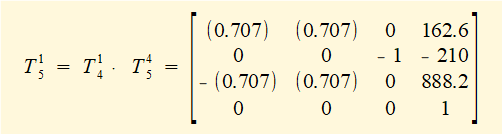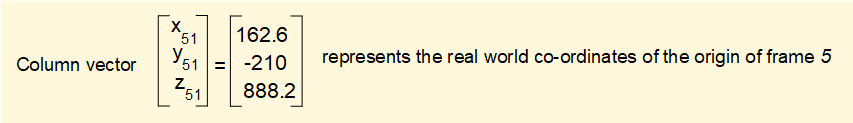Pair 5 - frame 4 and frame 5 (n = 5)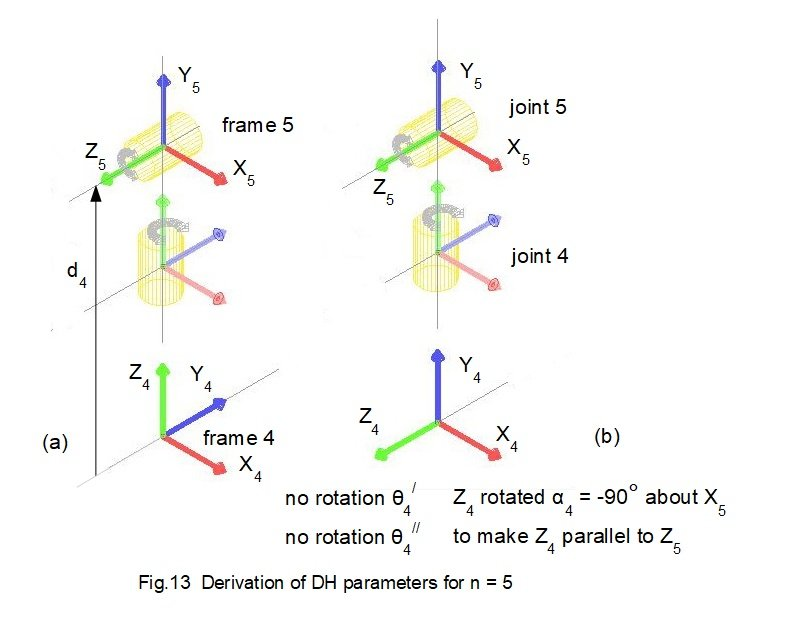Fig13(a) above shows frame 4 and frame 5 set up using the DH rules (as Fig.6).   Frame 5 is frame n and frame 4 is frame (n-1)  Consider each DH parameter in turn.

Parameter θ5

θ/5 is the angle required to rotate axis X4 around axis Z4  such that axis X4 is parallel to axis X. Fig.13(a) shows X4 is already parallel to X5.  Thus θ/5 = 0°.

θ//5  is the angle revolute joint 4 rotates around axis Z4   .  In this example θ//5 = -90°.

Thus  θ5 =  θ/5  +  θ//5  = -0° + (-90°) = -90°

Parameter α5

α5 is the angle required to rotate axis Z4 around axis X5  such that axis Z4 is parallel to axis Z5 .   Fig.13(b) shows Z4 must be rotated 90° clockwise around X5 to be parallel to Z5.  Thus α5 = -90°.

Parameter r5

r5 is the displacement in the X4 direction from the centre of frame 4 to the centre of frame 5 as shown on Fig.13(a).  In this case r5 = 0.

Parameter d5

d5 is the displacement in the Z4 direction from the centre of frame 4 to the centre of frame 5 as shown on Fig13(a).   From the robot model, d5 = 220.

The fifth row of the DH parameter table is now complete.

 Frames n θ α r d 0, 1 1 0° 90° 0 160 1, 2 2 135° 0° 400 135 2, 3 3 -180° -90° 0 75 3, 4 4 0° 90° 0 630 4, 5 5 -90° -90° 0 220

We compute the DH transformation matrix T45 to give: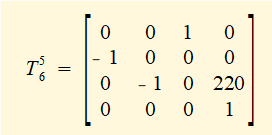and then compute T05 = T04 . T45 giving: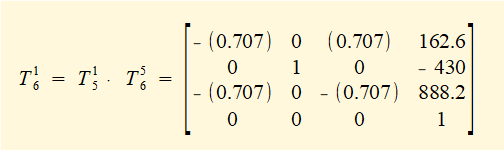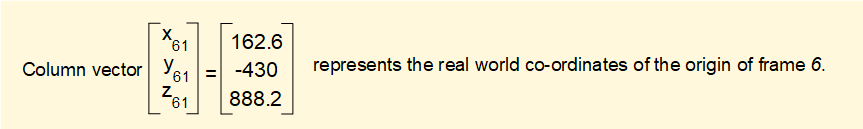Pair 6 - frame 5 and frame 6 (n = 6)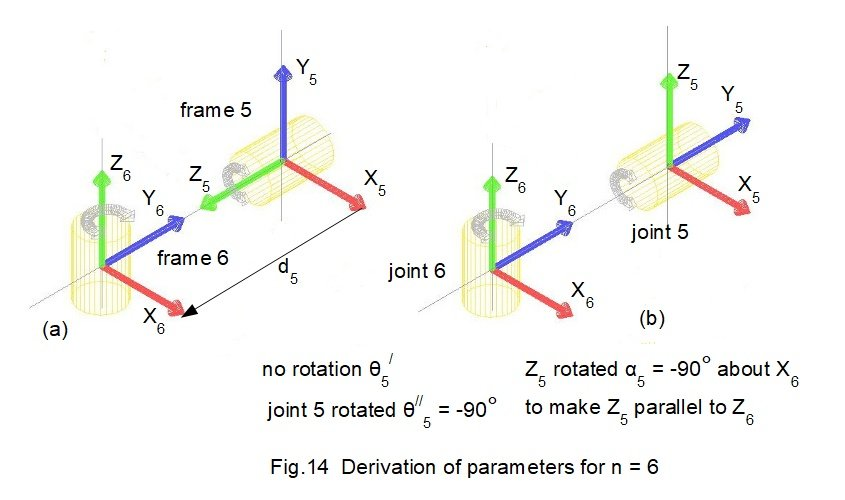Fig.14(a) above shows frame 5 and frame 6 (tool tip) set up using the DH rules (as Fig.6).   Frame 6 is frame n and frame 5 is frame (n-1)  Consider each DH parameter in turn.

Parameter θ6

θ/6 is the angle required to rotate axis X5 around axis Z5  such that axis X5 is parallel to axis X. Fig.14(a) shows X5 is already parallel to X6.  Thus θ/6 = 0°.

θ//6  is the angle revolute joint 5 rotates around axis Z5   .  In this example θ//6 = 0°.

Thus  θ6 =  θ/6  +  θ//6  = 0° + 0° = 0°

Parameter α5

α6 is the angle required to rotate axis Z5 around axis X6  such that axis Z5 is parallel to axis Z6 .   Fig.14(a) shows Z5 is already parallel to Z6.    Thus α6 = 0°.

Parameter r6

r6 is the displacement in the X5 direction from the centre of frame 5 to the centre of frame 6 as shown on Fig.14(a).  In this case r6 = 0.

Parameter d6

d6 is the displacement in the Z5 direction from the centre of frame 5 to the centre of frame 6 as shown on Fig14(a).   From the robot model, d6 = 110.

The sixth and final row of the DH parameter table is now complete.

 Frames n θ α r d 0, 1 1 0° 90° 0 160 1, 2 2 135° 0° 400 135 2, 3 3 -180° -90° 0 75 3, 4 4 0° 90° 0 630 4, 5 5 -90° -90° 0 220 5, 6 6 0° 0° 0 110

We compute the DH transformation matrix T56 to give: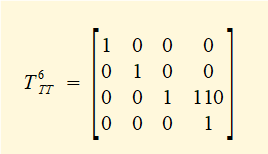and then compute T06 = T05 . T56 giving: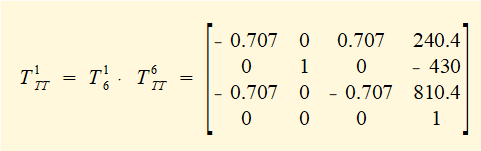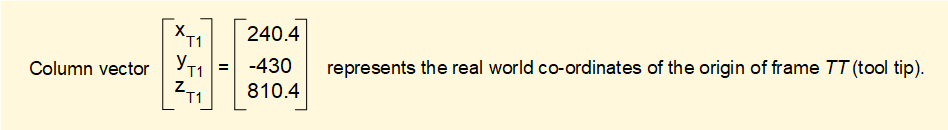And the marathon Denavit Hartenberg analysis is complete!

The real world co-ordinates computed for each frame correspond exactly to the values in the CAD drawing of the robot model.

I welcome feedback at:

### Tutorials - mechanical vibrations

###### Forced vibrations with damping

Alistair's tutorials 2021